8. Isoperimetric problems.

Problem: Minimize the functionalunder the constraintwhereMethod: In principle we follow the procedure from part 8 in the chapter about calculus of variations. We look for a local minimum y(x) in a family of admissible functions. However, we cannot use a one-parameter familylike in chapter 3, since a change of the parameter in general would have implied that the value of the constraint W(y) would have changed. We instead introduce a two-parameter familywhereand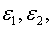are real parameters from an interval containing origo. We swap yfor z in the functionals and construct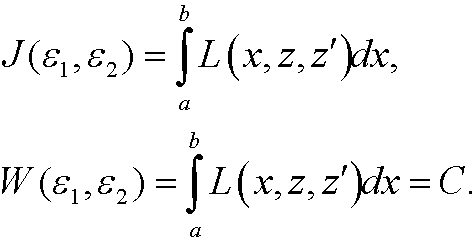Obviously, these parameters are not independent, since W(y) must be a constant C we have the relationbetween them. Since y(x) is an extremal, we must have an extreme value on J when. This is simply an extreme value problem with constraints, of the same type as we investigated in the previous part. We thus construct the function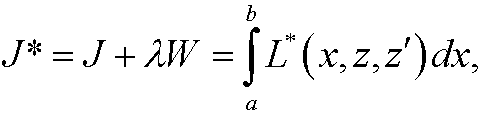whereAccording to the theorem in the previous part we then have the condition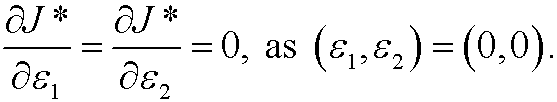These derivatives are equal to (by the chain rule)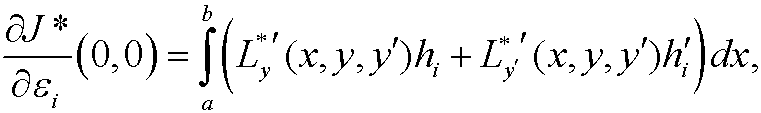for i=1,2We integrate by parts and use the boundary conditions on h1 and h2 and getfor i=1,2. With the lemma from part 8 in chapter 3 and the fact that h1 and h2 were arbitrary functions we get that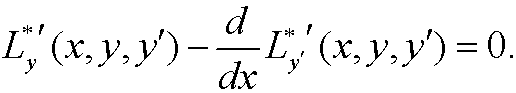A necessary condition for minimum (extremum) is thus that L* fulfills Euler-Lagrange’s equation

Remark 1: The problem and its method of solution can be generilzed to higher dimensions and more constraints in a straightforward way.

Remark 2: The solution we get from Euler-Lagrange’s equation above often depend on two unknown constants and the multiplier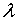. These can be determined by help of the boundary conditions and the constraint.